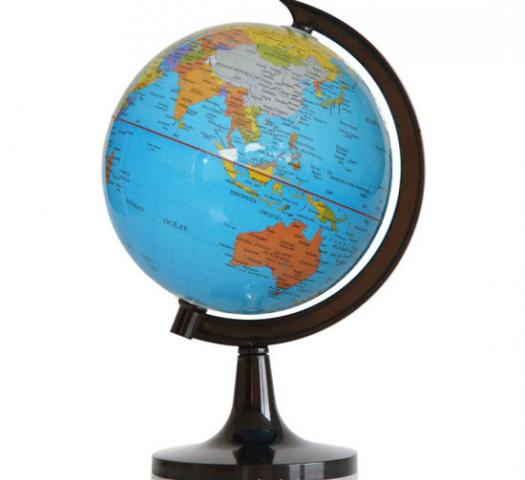# The Globe-a Model Of The Earth

10 Questions | Total Attempts: 54SettingsAnswer the following questions. Each question carries 1 point.

• 1.
The Earth rotates along an imaginary line called the
• A.

Axis

• B.

Equator

• C.

Meridian

• D.

Hemisphere

• 2.
The two end points of the axis of rotation are called
• A.

Parallels

• B.

Meridians

• C.

Poles

• D.

Hemisphere

• 3.
The shape of our planet Earth is
• A.

Oval

• B.

Spherical

• C.

Triangular

• D.

Cone

• 4.
A small model of the Earth which shows us its different features on a smaller scale.
• A.

Equator

• B.

Northern Hemisphere

• C.

Globe

• D.

Southern Hemisphere

• 5.
The large water bodies on the Globe are shown in which colour.
• A.

Brown

• B.

Black

• C.

White

• D.

Blue

• 6.
Large extensive masses of land on the Earth are called as
• A.

Oceans

• B.

Continents

• C.

Planets

• D.

Jupiter

• 7.
Two important tools to study the Earth are
• A.

Planets

• B.

The Sun

• C.

Globe and Maps

• D.

The Universe

• 8.
The imaginary line which divides the Earth into two equal halves is called the
• A.

Greenwich Meridian

• B.

Arctic Circle

• C.

Equator

• D.

Antarctic Circle

• 9.
The Globe helps us to show.(Mark any three options)
• A.

Shows exact location

• B.

Shows shapes of different features

• C.

Shows the animals

• D.

Gives an idea of the relative size of the land areas and water bodies .

• 10.
Does the planet Earth appear blue in colour from space( true or false?)
• A.

True

• B.

FalseBack to top